# Introduction to Reinforcement Learning

0
3786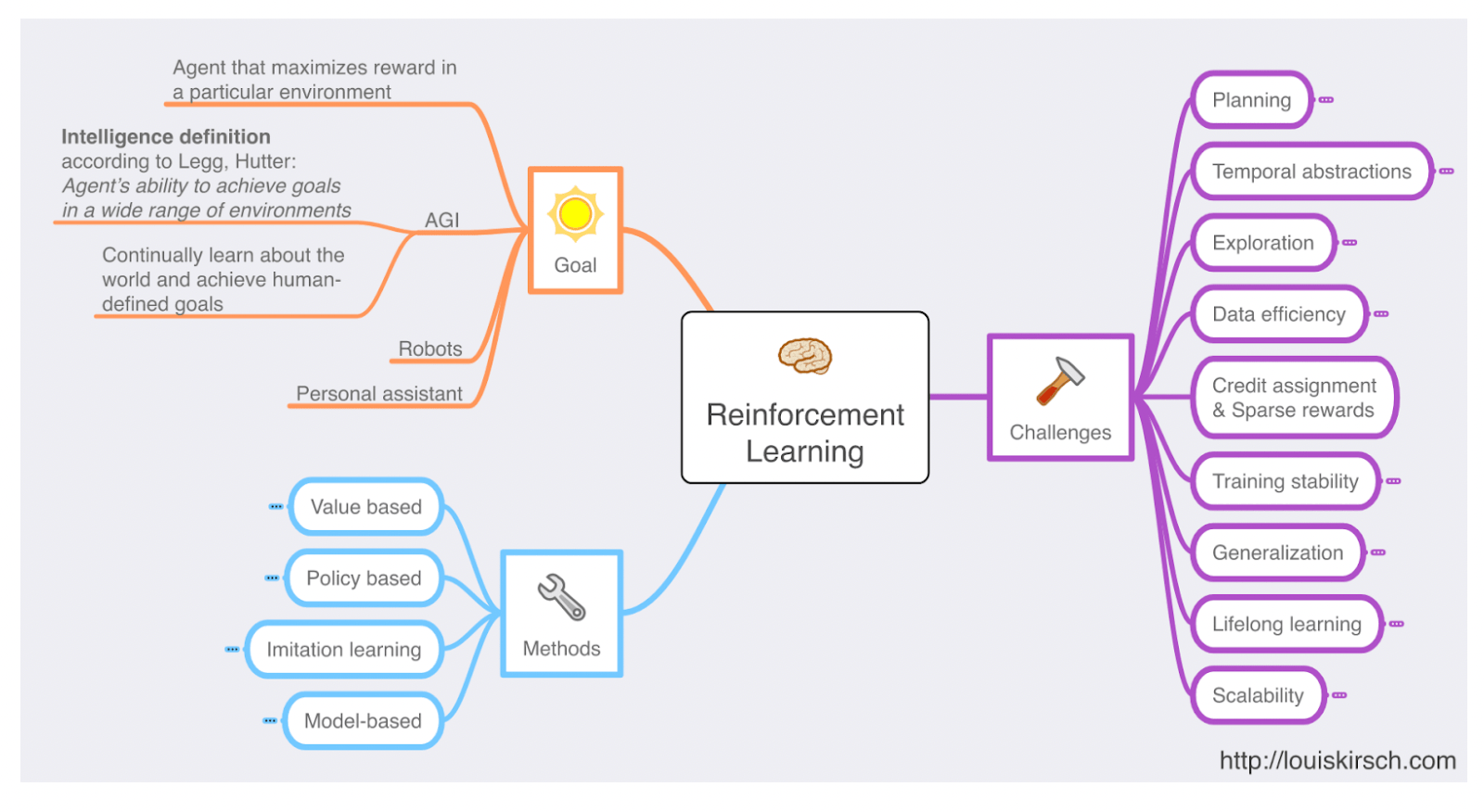source:louiskirsch.com

Reinforcement learning is a field of machine learning wherein the goal is learning to perform specific actions in an environment which leads to finding the right actions to be taken in different situations to achieve the absolute goal. The Agent learns to achieve a goal in an uncertain, complex environment through repeated trials to maximize a cumulative reward. The Agent employs a trial and error methodology to come up with a solution to the problem and gets either rewards or penalties for the actions it performs. Reinforcement Learning is employed to find the best possible behavior or path that should be taken in a given situation.

Reinforcement learning differs from supervised learning in not needing labeled input/output pairs to be presented to make the model learn something. In reinforcement learning, there is no answer, but the reinforcement agent decides what to do to perform the given task. In the absence of a training dataset, the focus is on finding a balance between exploration, exploitation, and learning from experience.

The essential elements of a Reinforcement learning model

The Agent is the learner and decision-maker of the model to do the job specified. The Environment is the world with which the Agent interacts and performs actions based on the information it has learned. The Agent receives state S⁰ from the Environment, and subsequently, the Agent and the Environment interact continually. The Agent selects actions, and the Environment responds to those actions and presents new situations to the Agent.

Action is a move made by the Agent, which causes a status change in the Environment. The Agent can take any random action and move to a new state. The best action is decided based on the maximum reward. A reward is the evaluation of an action given by the Environment and can be positive or negative. The fundamental goal of our model is to select actions to maximize the total reward.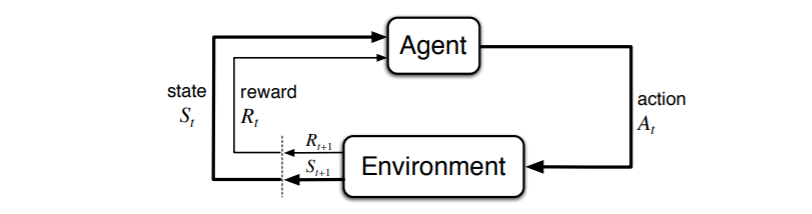source: Stanford-edu Docs

Approaches to a Reinforcement Learning Problem

A reinforcement learning problem can be approached in many ways:

There is no policy function in a value-based reinforcement learning approach, and the aim is to greedily select actions to maximize a value function V(s).

In a policy-based approach, the action performed at each state to gain maximum reward in the future is based on a policy function. Here, no value function is involved. The policy function can be Deterministic, which produces the same action A at any state s, or Stochastic, where each action A has a certain probability of occurrence.

A Model-Based approach generates a virtual model for each Environment, and the Agent learns to produce actions in that specific Environment. Since the model differs for each Environment, there is no singular solution or algorithm for this approach.

Implementing a Reinforcement Learning model

OpenAI Gym is a framework for developing reinforcement learning algorithms.OpenAI Gym provides various game environments in which the Agent can take action. Each Environment has an initial status, and the status is updated each time the Agent takes action.

Here we will be implementing our reinforcement learning model on the cart pole game from OpenAI and creating a high score!!

Write the following code to implement the model:

``````#importing essential libraries
import gym
import numpy as np
import matplotlib.pyplot as plt
import os
from collections import deque
from keras.models import Sequential
from keras.layers import Dense
from keras.optimizers import Adam
import random

#Creating Agent
class Agent:
def __init__(self,state_size,action_size):
self.state_size = state_size
self.action_size = action_size
self.memory = deque(maxlen=2000)
self.gamma = 0.95 #Discount Factor
self.epsilon = 1.0 # Exploration Rate: How much to act randomly,
self.epsilon_decay = 0.995
self.epsilon_min = 0.01
self.learning_rate = 0.001
self.model = self._create_model()

#Creating Model
def _create_model(self):
#Neural Network To Approximate Q-Value function
model = Sequential()
#1st Hidden Layer
model.add(Dense(24,input_dim=self.state_size,activation='relu'))
model.add(Dense(24,activation='relu')) #2nd Hidden Layer
model.add(Dense(self.action_size,activation='linear'))
model.compile(loss='mse',optimizer=Adam(lr=self.learning_rate))
return model

#remembering previous experiences
def remember(self,state,action,reward,next_state,done):
self.memory.append((state,action,reward,next_state,done))

#Creating an action function
def act(self,state):
# Exploration vs Exploitation
if np.random.rand()<=self.epsilon:
return random.randrange(self.action_size)
# predict reward value based upon current state
act_values = self.model.predict(state)
return np.argmax(act_values) #Left or Right

#method that trains NN with experiences sampled from memory
def train(self,batch_size=32):
minibatch = random.sample(self.memory,batch_size)
for state,action,reward,next_state,done in minibatch:

if not done: #boolean
target = reward + self.gamma*np.amax(self.model.predict(next_state))
else:
target = reward
target_f = self.model.predict(state)
target_f[action] = target
self.model.fit(state,target_f,epochs=1,verbose=0)

if self.epsilon > self.epsilon_min:
self.epsilon *= self.epsilon_decay

def load(self,name):
self.model.load_weights(name)
def save(self,name):
self.model.save_weights(name)

#Running the model
n_episodes = 1000
output_dir = "cartpole_model/"
agent = Agent(state_size=4,action_size=2)
done = False
state_size = 4
action_size =2
batch_size = 32
agent = Agent(state_size, action_size) # initialise agent
done = False
for e in range(n_episodes):
state = env.reset()
state = np.reshape(state,[1,state_size])

for time in range(5000):
env.render()
action = agent.act(state) #action is 0 or 1
next_state,reward,done,other_info = env.step(action)
reward = reward if not done else -10
next_state = np.reshape(next_state,[1,state_size])
agent.remember(state,action,reward,next_state,done)
state = next_state

if done:
print("Game Episode :{}/{}, High Score:{},Exploration Rate:{:.2}".format(e,n_episodes,time,agent.epsilon))
break

if len(agent.memory)>batch_size:
agent.train(batch_size)

if e%50==0:
agent.save(output_dir+"weights_"+'{:04d}'.format(e)+".hdf5")

env.close()
``````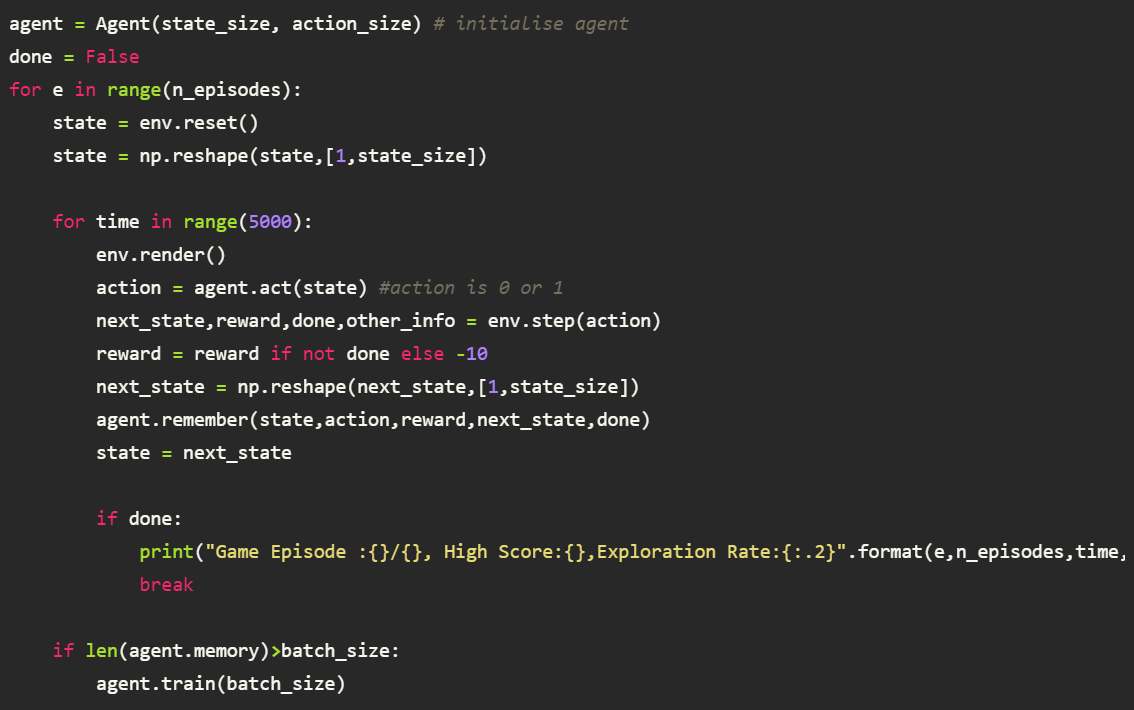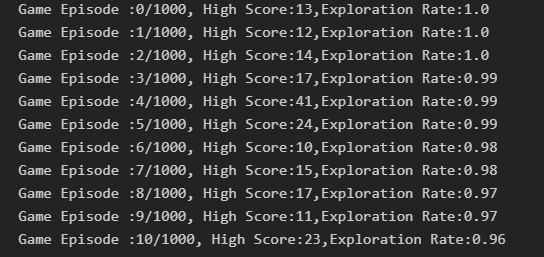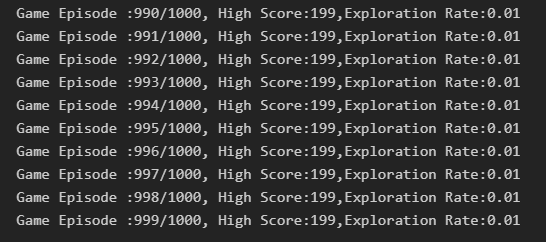Visit the OpenAI Gym to find many exciting environments to work on and know more about Reinforcement Learning.

Happy Learning!!

This site uses Akismet to reduce spam. Learn how your comment data is processed.